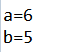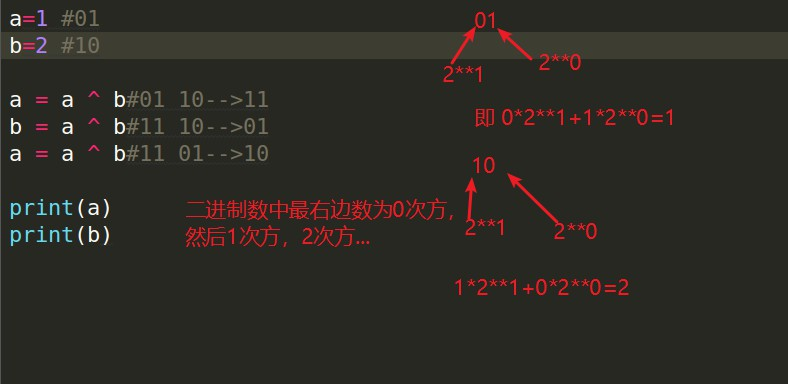• 最近在写位操作的时候突然想到了这问题，突然想总结一下，交换变量值的问题可能使我们学习编程语言接触到的比较早的逻辑问题，小伙伴或多或少会种解决的方法，本文提供了七种解决的方法，下面跟着小编来一起看看...
• 交换两个变量 int a = 10; int b = 20; 方案A:使用第三方变量（开发中使用） int c = a; a = b; b = c; 方案B:用异或的特点实现（面试使用） a = a ^ b; b = a ^ b;//a ^ b ^ b = a a = a ^ b;//a ^ b ^ a = b ...
面试题： 交换两个变量的值
int a = 10;
int b = 20;

方案A:使用第三方变量（开发中使用）
int c = a;
a = b;
b = c;
方案B:用异或的特点实现（面试使用）
a = a ^ b;
b = a ^ b;//a ^ b ^ b = a
a = a ^ b;//a ^ b ^ a = b
方案C:用变量相加的方法
a = a + b;
b = a - b;
a = a - b;
方案D:
b = (a + b) - (a = b);
展开全文• 在日常编程里面经常会遇到交换两个变量的内容的任务，对于泛型类型而言有两种泛型策略来实现，下面跟着小编一起来学习学习。
• 1.加减法 #include <stdio.h> int main(int argc, char *argv[]) { int a=1,b=2; a=a+b;...适用于交换整型变量，浮点型变量交换可能会损失精度。2.乘除法 #include <stdio.h> i...
1.加减法
#include <stdio.h>
int main(int argc, char *argv[])
{
int a=1,b=2;
a=a+b;
b=a-b;
a=a-b;
printf("a=%d b=%d",a,b);
}

适用于交换整型变量，浮点型变量交换可能会损失精度。2.乘除法
#include <stdio.h>
int main(int argc, char *argv[])
{
int a=1,b=2;
a = a * b;
b = a / b;
a = a / b;
printf("a=%d b=%d",a,b);
}
其中a, b必须为非0值，适用于交换整型变量，浮点型变量交换可能会损失精度。3.异或法
#include <stdio.h>
int main(int argc, char *argv[])
{
int a=1,b=2;
a ^= b;
b ^= a;
a ^= b;
printf("a=%d b=%d",a,b);
}
只适用于对整型变量进行交换，不适用于浮点型变量交换。
4.强制转换移位法
#include <stdio.h>
int main(int argc, char *argv[])
{
int a=1,b=2;
b = (long int)((long int)a << 32 | (a = b)) >> 32;
printf("a=%d b=%d",a,b);
}
只适用于对整型变量进行交换，不适用于浮点型变量交换。
展开全文• 今天小编就为大家分享一篇python交换两个变量方法，具有很好的参考价值，希望对大家有所帮助。一起跟随小编过来看看吧
• method 1： a，b = b，a method 2： a = a+b b = a-b ... 您可能感兴趣的文章:python交换两个变量方法对Python中实现两数的交换的集中方法详解对变量赋值的理解–Pyton中让两个值互换的实现方法
• 内容索引:Delphi源码,算法相关,变量,算法　在前人的基础上修改的，交换两个变量值的算法，望高手能找出比这更好的解决方法，一起提高。
• Java 交换两个变量值的方法 第一种：使用第三变量进行交换 int a = 5; int b = 6; int c = a; a = b; b = c; 输出结果截图： 第二种：不使用第三变量（利用加法） int a = 5; int b = 6; a = a +...
Java 交换两个变量值的方法

第一种：使用第三个变量进行交换
int a = 5;
int b = 6;
int c = a;
a = b;
b = c;
输出结果截图：第二种：不使用第三个变量（利用加法）
int a = 5;
int b = 6;
a = a + b;
b = a - b;
a = a - b;
结果：展开全文Java Java基础
• python中交换两个变量值的几种方式 1.python中独有的，也是最简单的方式： a,b=b,a 2.引入第三变量，可以看作两装满牛奶的瓶子a和瓶子b，如果我想交换这两瓶子中的牛奶，就需要一空瓶temp，先将a瓶中的...
python中交换两个变量值的几种方式
1.python中独有的，也是最简单的方式：
a,b=b,a
2.引入第三个变量，可以看作两个装满牛奶的瓶子a和瓶子b，如果我想交换这两个瓶子中的牛奶，就需要一个空瓶temp，先将a瓶中的牛奶倒入空瓶temp中，此时a瓶为空，可以将b瓶中的牛奶倒入a中，然后再将temp中的牛奶倒入b中，这样就实现了交换。
temp=a
a=b
b=temp
3.采用特殊的运算方式：
a=1
b=2

a=a+b #1+2=3
b=a-b #3-2=1
a=a-b #3-1=2
4.使用python中位运算中异或^进行操作：
a=1 #转换成二进制数为01
b=2 #转换成二进制数为10

a = a ^ b #01^10-->11
b = a ^ b #11^10-->01
a = a ^ b #11^01-->10
异或操作是先将两个数转换成对应的二进制数，然后对两个二进制数进行按位异或，即两个数字不同则为1，否则为0。如1^0=1,0^0=0。展开全文• 在考试和面试时经常碰见交换两个变量，下面我就罗列出Python 语言交换两个变量的4种方法 方法1： ""常规方法，采用第三方变量"" x=2 y=3 z= x # z=2 x = y # x = 3 y=z # y =...
• 下面小编就为大家带来一篇Java 交换两个变量的数值实现方法。小编觉得挺不错的，现在就分享给大家，也给大家做参考。一起跟随小编过来看看吧
• //使用三种方法去交换两个变量的数值public class ExchangeTwoVariable { public static void main(String[] args) { //方法一（简单）： /* int a = 1,b = 2; int temp; System.out.println("交换前：a = " + a +java
• Javascript交换两个变量值的两种常见方式： 1.借助于第三方变量的方式交换变量值 然后console.log在控制台中输出，num1与num2成功完成交换 2.第二种变量交换方式，一般适用于数字交换 ...javascript
• ## C++中交换两个变量的值的方法

千次阅读 多人点赞 2019-11-05 18:21:03
即不能通过将变量名传入函数的方式进行交换，因为这种方式是将进行拷贝后作为参数传入函数，也就是说函数内部使用的拷贝的，在函数执行完后就会销毁，而不是对变量的原值进行操作。 而在C++中需要通...
• 两个变量怎么交换值 1.通常的办法是设一临时变量tmp，将两个值进行交换 2.但这里有有趣的方法是利用位运算进行交换值的操作。 首先有原理需要知道： 若a^b=c,则c^b=a,c^a=b 可通过穷举法进行证明 那么...位运算 算法
• 下面给大家推荐一经典面试题：交换两个变量，不使用其他变量交换两个变量，最初想到的就是利用第三个变量，so easy。当不用第三方变量时，怎么交换呢？ 题目：交换两个变量，不使用其他变量 a = 6 ...
• 在一些公司的面试中可能会有些面试官会出一些奇奇怪怪的问题，比如怎么样计算1加到100的和要求不能使用加法，减法，循环和if判断，再比如怎么样不用第三个变量交换两个变量等问题。这里我们先分析下怎么样不用...C++
•   谈到算法，好多人都感到脑壳疼，我也不例外。主要是平时工作忙，下班了还有各种进步，基本对算法这一块研究的较少。...题目：实现交换两个变量 可以实现的算法如下： 1，借助中间变量进行交换 //借助中...算法 空间 时间 C语言
• SWAP(A,B) 将变量 A 的内容放入变量 B，反之亦然。 您可以使用函数语法'swap（A，B）'或命令语法'swap A B'。 例子： A = 1:4 ;... 与这种方法相比的优势在于，使用 SWAP 一种不必声明中间变量或担心输出。matlab
• 在学习.Net/C#或者任何一门面向对象语言的初期，大家都写过交换两个变量值，通常是通过临时变量来实现。本篇使用多种方式实现两个变量值的交换。 假设int x =1; int y = 2;现在交换两个变量的值。 使用临时变量实现 ...
• 面试中经常考到的一题。原理跟 a=a+b;...//宏定义 交换两个变量。使用异或运算。 #define SWAP(a,b) {a=a^b; b=a^b; a=a^b;} int main() {  int i, j;  i = 1213;  j = 1314;  SWAP(i, j);  ...C语言
• 第二种方法就是不使用第三变量的情况下交换两个变量值。 下面我们就通过具体的代码示例，给大家介绍PHP交换两个变量值的方法。 首先大家要知道交换两个变量就是指相互交换变量的值。通常，这是通过内存中的数据...
• ## 交换两个变量的值的三种方法

千次阅读 多人点赞 2021-03-06 19:04:20
方法一：定义临时变量法 运用此方法比较简单 public class 定义临时变量 { public static void main(String[] args) { int v1=1; int v2=2; System.out.println("v1="+v1+","+"v2=...//实现交换 v1=v2; v2=tempjava
• 在go之前的编程语言中，如果要交换变量，需要借助一临时变量来实现。如下伪代码： 变量三 = 变量变量一 = 变量变量二 = 变量三 go语言提供了多重赋值的特性可以轻松实现变量交换。如下伪代码： 变量...
• 给两整型变量赋值，然后交换两个变量的值，最后输出交换后的变量值
• ## Java中三种方法交换两个变量的值

千次阅读 多人点赞 2019-07-21 19:09:57
1、中间变量 是最普也是最广泛使用的交换方式： ...第一步求出数的和为10，传给m，再用总数减去n就是原来m的，传给n，然后总数再减去原来m的，得到原来n的，传给m，实现交换。 3.异或运...java
•  相比使用一局部变量交换两个变量，这种方法并没有性能上的优势，只是一种对位运算的练习。   一数与它本身异或，结果为0。 假设*x = a；*y = b； *x *y Step0 a b Step1......